Ch 1. Stress and Strain Multimedia Engineering Mechanics NormalStress Shear andBearing Stress NormalStrain Hooke'sLaw ThermalEffects IndeterminateStructures
 Chapter 1. Stress/Strain 2. Torsion 3. Beam Shr/Moment 4. Beam Stresses 5. Beam Deflections 6. Beam-Advanced 7. Stress Analysis 8. Strain Analysis 9. Columns Appendix Basic Math Units Basic Equations Sections Material Properties Structural Shapes Beam Equations Search eBooks Dynamics Fluids Math Mechanics Statics Thermodynamics Author(s): Kurt Gramoll ©Kurt GramollMECHANICS - THEORY

Shear Stress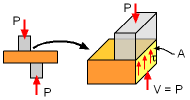In addition to normal stress that was covered in the previous section, shear stress is an important form of stress that needs to be understood and calculated. Most structures need to be designed for both normal and shear stress limits.

Similar to average normal stress (σ = P/A), the average shear stress is defined as the the shear load divided by the area.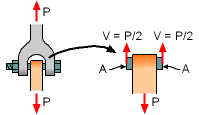τ = V/A

Shear loads are generally identified by the symbol V and shear stress by the greek symbol tau, τ. However, this is not a fixed rule.

Another way to express the same concept is to define the total surface parallel load V as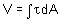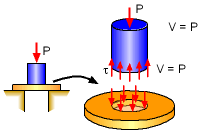where A is the area of the surface.

One confusing issue with shear stress is direction. Unlike normal stress with compression or tension, shear stress is the same if it shears left to right or right to left. The magnitude of shear stress is the important parameter that needs to be considered.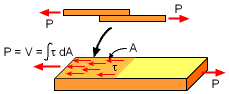It is important to remember that V/A is only the "average" shear stress. In actual shear stress distribution, it is not uniform and the maximum shear stress will be higher than the average shear stress. However, for this basic solid mechanics course, it is assumed that the shear load is uniformly distributed across the shear surface.

Bearing Stress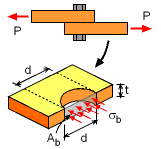Bearing Stress Due to a Bolt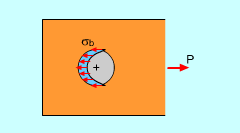Actual Bearing Stress on Plate

The average bearing stress is the force pushing against a structure divided by the area. Exact bearing stress is more complicated but for most applications, the following equation works well for the average,

 σb = P/Ab

This relationship can be further refined by using the width and height of the bearing area as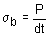Experiments have shown that the bearing stress distribution is more elliptical shaped and not uniform but due to calculation difficulties, a rectangular approximation is assumed, dt.

Practice Homework and Test problems now available in the 'Eng Mechanics' mobile app
Includes over 400 problems with complete detailed solutions.
Available now at the Google Play Store and Apple App Store.